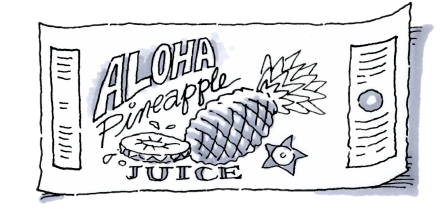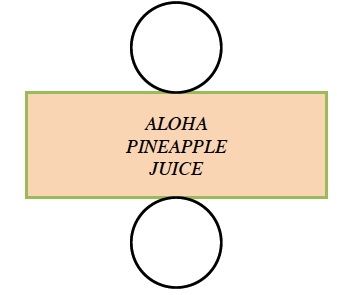### Home > CC2MN > Chapter 11 > Lesson 11.1.5 > Problem11-68

11-68.

The label of the Aloha Pineapple Juice can is $7$ inches high and has an area of $140$ square inches. What is the diameter of the can?The label wraps around a cylinder.
It may be useful to draw a net of this cylinder.You know the equation for the area of a rectangle can be found using the formula:
$\text{Area = (base)(height)}$
Substitute the known values and solve for the unknown value.

$140 = (\text{base})(7)$

$(\frac{140}{7})=\text{base}(\frac{7}{7})$

$\text{base} = 20$ inches

You learned that the width of the rectangle is equal to the circumference of the circle. Substitute the known values into the following equation and solve for the diameter:
$\text{Circumference = (diameter)}π$

$20 = \text{(diameter)} · π$

$\frac{20}{\pi }=(\text{diameter})\frac{\pi }{\pi }$

Diameter = ?